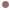Autres Articles de :
Algorithms

# Fitting polynomials for computing time derivatives

Christophe LETELLIER
13/03/2008Motivations

As soon as derivatives estimated from experimental data are involved, comes the question whether the contaminating noise is a problem to safely compute derivatives from such data. The answer depends on the way derivatives are estimated. Using Euler scheme or finite difference scheme leads to a negative answer, mainly because the noise contamination is very quickly amplified from one derivative to the other. Using Legendre polynomials as discussed in  provides slightly better results but, we observed a significant improvement in the use of derivative coordinates when locally fitted polynomials were analytically derived. Such a technique presents the great advantage to spread the noise contribution in a homogeneous way in all the coordinates. Moreover, successive derivatives share a coherence that other methods do not provide. For instance, in the case of Legendre polynomials, each derivative is estimated independently, a property not recommended for global modelling.Algorithm for computing time derivativesThe algorithm

The central idea of our technique to compute derivatives is to use local interpolating polynomials to compute the derivatives instead of computing the derivatives directly from the data. To compute the derivatives throughout the whole time series, a moving window method is employed. The procedure can be summarized as follows:

• Choose a windowof lenghtin the beginning of the time series, that is,;
• Find an interpolating polynomialof degreethat passes through all the points of;
• Compute the derivatives at the center of the windowby analytically derivating the polynomial at this point;
• Move the window one sample forward in time and repeat all the steps until all the time series have been processed.
• There is a low-pass filter in a frequency domain (function smooth). The filtering parameter (vois) is expressed in terms of number of data points. It should be taken as small as possible.

This method was never published before this recent paper about Frequently Asked Questions about global modelling .An example

The space reconstructed spanned by the successive time derivatives computed from the anode currentrecorded in the copper electrodissolution experiments is here shown. It was produced with the Fortran code you can download from this page with the parameters left in the code. In fact the model we got from this experiments was obtained with derivative using Legendre polynomials . This is only later that Loïs Le Sceller developed this procedure to compute derivatives . Then, this was applied to a velocity component of the flow in a mixing tank , a Belousov-Zhabotinsky reaction   and the Lynx population .Differential space from the copper electrodissolution

 J. F. Gibson, J. D. Farmer, M. Casdagli & S. Eubank, An analytic approach to practical state space reconstruction, Physica D, 57, 1-30, 1992.

 C. Letellier, L. A. Aguirre & U. S. Freitas, Frequently Asked Questions about global modelling, submitted.

 C. Letellier, L. Le Sceller, E. Maréchal, P. Dutertre, B. Maheu, G. Gouesbet, Z. Fei & J. L. Hudson, Global vector field reconstruction from a chaotic experimental signal in copper electrodissolution, Physical Review E, 51 (5), 4262-4266, 1995.

 L. Le Sceller, Reconstruction globale de champ de vecteurs et Applications, Ph’D Thesis defended on February 28, 1997 at CORIA UMR 6614, Université de Rouen.

 C. Letellier, L. Le Sceller, G. Gouesbet, F. Lusseyran, A. Kemoun & B. Izrar, Recovering deterministic behavior from experimental time series in a standard mixing reactor, AIChE Journal, 43 (9), 2194-2202, 1997.

 C. Letellier, J. Maquet, H. Labro, L. Le Sceller, G. Gouesbet, F. Argoul & A. Arn\’eodo, Analyzing chaotic behaviour in a Belousov-Zhabotinskii reaction by using a global vector field reconstruction, Journal of Physical Chemistry A, 102, 10265-10273, 1998.

 J. Maquet, C. Letellier & L. A. Aguirre, Global models from the Canadian Lynx cycles as a first evidence for chaos in real ecosystems, Journal of Mathematical Biology, 55 (1), 21-39, 2007.

### Documents

Algorithm for computing time derivativesZip · 3.7 kb
12513 - 19/09/21